# Overall Survival Curves for TCGA and Tothill by RD Status

by Susan L. Tucker

``````opts_chunk\$set(tidy = TRUE, message = TRUE)
``````

## 1 Executive Summary

### 1.1 Introduction

The goal of this analysis is to produce Kaplan-Meier curves of overall survival (OS) by residual disease (RD) status for patients included in TCGA and Tothill et al.

### 1.2 Data & Methods

We use the RData objects containing clinical information created in previous reports (assembleTCGAClinical, assembleTothillClinical). Patients are filtered as described previously (filterTCGASamples, filterTothillSamples). Additional patients are excluded for whom survival information is missing.

Survival times are converted from months to years for the data of Tothill et al.

Kaplan-Meier plots are produced to illustrate OS in patient cohorts. OS is compared between groups using the log-rank test.

Comparisons considered are:

i) TCGA versus Tothill et al.

ii) Within each dataset by the RD categories provided in the original data sources.

iii) Within each dataset, any RD compared to no RD.

iv) Within each dataset, by FABP4 expression.

### 1.3 Results

Three patients are excluded from the filtered cohort of Tothill et al. because of missing survival information.

OS is essentially identical in TCGA and Tothill et al.

Within each data set, OS differs significantly by RD status, using both the RD categories provided or comparing any RD to no RD.

In each data set, OS is worse among the 25% of patients with the highest expression levels of FABP4. The difference reaches statistical significance in Tothill et al.

``````

``````

Filtrations are applied to the TCGA data.

``````
rownames(tcgaFilteredSamples)[1:2]
``````
``````##  "TCGA-13-0758-01A-01R-0362-01" "TCGA-09-0364-01A-02R-0362-01"
``````
``````rownames(tcgaClinical)[1:2]
``````
``````##  "TCGA-04-1331" "TCGA-04-1332"
``````
``````rownames(tcgaOSYrs)[1:2]
``````
``````##  "TCGA-04-1331" "TCGA-04-1332"
``````
``````colnames(tcgaExpression[, 1:2])
``````
``````##  "TCGA-13-0758-01A-01R-0362-01" "TCGA-09-0364-01A-02R-0362-01"
``````
``````
tcgaSampleUseLong <- rownames(tcgaFilteredSamples[which(tcgaFilteredSamples[,
"sampleUse"] == "Used"), ])
tcgaSampleUse <- substr(tcgaSampleUseLong, 1, 12)
length(tcgaSampleUse)
``````
``````##  491
``````
``````length(unique(tcgaSampleUse))
``````
``````##  491
``````
``````
tcgaOSYrsUse <- tcgaOSYrs[tcgaSampleUse, ]
summary(tcgaOSYrsUse)
``````
``````##       time            status
##  Min.   : 0.025   Min.   :0.000
##  1st Qu.: 0.936   1st Qu.:0.000
##  Median : 2.323   Median :1.000
##  Mean   : 2.652   Mean   :0.532
##  3rd Qu.: 3.760   3rd Qu.:1.000
##  Max.   :12.666   Max.   :1.000
``````
``````tcgaClinUse <- tcgaClinical[tcgaSampleUse, ]
tcgaRDUse <- tcgaRD[tcgaSampleUse]
table(tcgaRDUse)
``````
``````## tcgaRDUse
## No RD    RD
##   113   378
``````
``````
tcgaExpressionUse <- tcgaExpression[, tcgaSampleUseLong]
colnames(tcgaExpressionUse) <- tcgaSampleUse
``````

Filtrations are applied to the data of Tothill et al. and survival times are converted from months to years.

``````
rownames(tothillFilteredSamples)[1:2]
``````
``````##  "X49"  "X129"
``````
``````rownames(tothillClinical)[1:2]
``````
``````##  "X49"  "X129"
``````
``````rownames(tothillOSMos)[1:2]
``````
``````##  "X49"  "X129"
``````
``````colnames(tothillExpression[, 1:2])
``````
``````##  "X60120" "X32117"
``````
``````
tothillSampleUseTmp <- rownames(tothillFilteredSamples[which(tothillFilteredSamples[,
"sampleUse"] == "Used"), ])
length(tothillSampleUseTmp)
``````
``````##  189
``````
``````summary(tothillOSMos[tothillSampleUseTmp, ])
``````
``````##       time           status
##  Min.   :  0.0   Min.   :0.000
##  1st Qu.: 18.0   1st Qu.:0.000
##  Median : 27.0   Median :0.000
##  Mean   : 30.7   Mean   :0.455
##  3rd Qu.: 41.0   3rd Qu.:1.000
##  Max.   :166.0   Max.   :1.000
##  NA's   :3
``````
``````
tothillSampleUse <- intersect(tothillSampleUseTmp, rownames(tothillOSMos[!is.na(tothillOSMos[,
1]), ]))
length(tothillSampleUse)
``````
``````##  186
``````
``````
tothillOSYrsUse <- tothillOSMos[tothillSampleUse, ]
tothillOSYrsUse[, 1] <- tothillOSYrsUse[, 1]/12
tothillClinUse <- tothillClinical[tothillSampleUse, ]
tothillRDUse <- tothillRD[tothillSampleUse]
table(tothillRDUse)
``````
``````## tothillRDUse
## No RD    RD
##    50   136
``````
``````
tothillExpressionUse <- tothillExpression[, tothillSampleUse]
``````

## 3 Analyses

Overall survival is compared in TCGA versus Tothill et al.

``````
tmp <- rbind(tcgaOSYrsUse, tothillOSYrsUse)

library(survival)
``````
``````## Loading required package: splines
``````
``````osAll <- Surv(tmp[, 1], tmp[, 2] == 1)

cohort <- rep(2, dim(osAll))
cohort[1:dim(tcgaOSYrsUse)] <- 1
table(cohort)
``````
``````## cohort
##   1   2
## 491 186
``````
``````
fit <- survfit(osAll ~ cohort)
survdiff(osAll ~ cohort)
``````
``````## Call:
## survdiff(formula = osAll ~ cohort)
##
##            N Observed Expected (O-E)^2/E (O-E)^2/V
## cohort=1 491      261    260.7  0.000242  0.000993
## cohort=2 186       86     86.3  0.000731  0.000993
##
##  Chisq= 0  on 1 degrees of freedom, p= 0.975
``````
``````
plot(fit, lty = c(1, 2), xlab = "Years", ylab = "Overall Survival", lwd = 2,
main = "Overall Survival in TCGA versus Tothill")
legend(x = 8, y = 0.95, legend = c("TCGA (N=491)", "Tothill (N=186)"), lty = c(1,
2), lwd = 2)
text(11, 0.7, "P = 0.975")
``````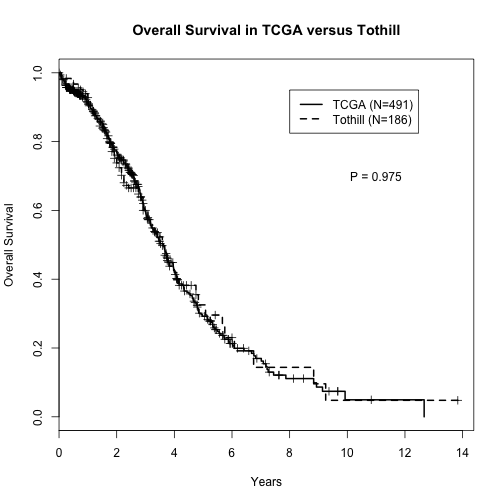Overall survival by residual disease status is plotted for the TCGA data.

``````
table(tcgaClinUse\$tumor_residual_disease)
``````
``````##
##        [Not Available]                 >20 mm                1-10 mm
##                      0                    102                    242
##               11-20 mm No Macroscopic disease
##                     34                    113
``````
``````tcgaGp <- rep(1, dim(tcgaOSYrsUse))
tcgaGp[which(tcgaClinUse[, "tumor_residual_disease"] == "1-10 mm")] <- 2
tcgaGp[which(tcgaClinUse[, "tumor_residual_disease"] == "11-20 mm")] <- 3
tcgaGp[which(tcgaClinUse[, "tumor_residual_disease"] == ">20 mm")] <- 4

survTCGA <- Surv(tcgaOSYrsUse[, 1], tcgaOSYrsUse[, 2] == 1)
tcgaSurvFit <- survfit(survTCGA ~ tcgaGp)
survdiff(survTCGA ~ tcgaGp)
``````
``````## Call:
## survdiff(formula = survTCGA ~ tcgaGp)
##
##            N Observed Expected (O-E)^2/E (O-E)^2/V
## tcgaGp=1 113       30     59.9    14.951    19.507
## tcgaGp=2 242      147    131.5     1.830     3.726
## tcgaGp=3  34       23     20.6     0.281     0.306
## tcgaGp=4 102       61     49.0     2.949     3.647
##
##  Chisq= 20.1  on 3 degrees of freedom, p= 0.000162
``````
``````
plot(tcgaSurvFit, lty = 1:4, xlab = "Years after Surgery", ylab = "Proportion Surviving",
lwd = 2)
legend(x = 5, y = 0.98, legend = c("No macroscopic disease (N=113)", "1-10 mm (N=242)",
"11-20 mm (N=34)", ">20 mm (N=102)"), lty = c(1:4), lwd = 2, cex = 0.8)
text(0.05, 0.05, "(A) TCGA", pos = 4)
text(7, 0.6, "P = 0.0002", pos = 4)
``````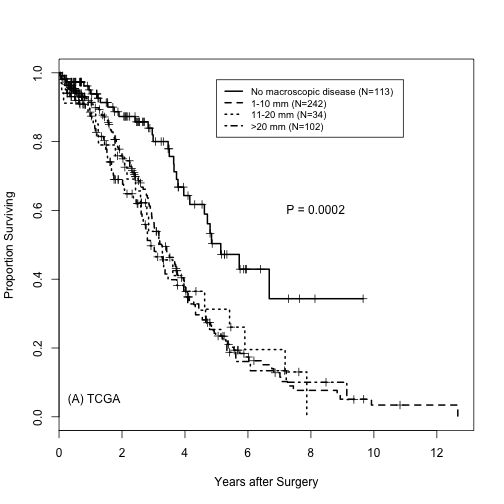Overall survival by residual disease status is plotted for the data of Tothill et al.

``````
table(tothillClinUse\$ResidDisease)
``````
``````##
##            <1            >1 macro size NK           nil            NK
##            66            57            13            50             0
``````
``````tothillGp <- rep(1, dim(tothillOSYrsUse))
tothillGp[which(tothillClinUse[, "ResidDisease"] == "<1")] <- 2
tothillGp[which(tothillClinUse[, "ResidDisease"] == ">1")] <- 3
tothillGp[which(tothillClinUse[, "ResidDisease"] == "macro size NK")] <- 4

survTothill <- Surv(tothillOSYrsUse[, 1], tothillOSYrsUse[, 2] == 1)
tothillSurvFit <- survfit(survTothill ~ tothillGp)
survdiff(survTothill ~ tothillGp)
``````
``````## Call:
## survdiff(formula = survTothill ~ tothillGp)
##
##              N Observed Expected (O-E)^2/E (O-E)^2/V
## tothillGp=1 50       14    26.97   6.23891   9.36475
## tothillGp=2 66       34    27.17   1.71681   2.64353
## tothillGp=3 57       31    25.10   1.38667   2.00101
## tothillGp=4 13        7     6.76   0.00872   0.00971
##
##  Chisq= 9.7  on 3 degrees of freedom, p= 0.0217
``````
``````
plot(tothillSurvFit, lty = 1:4, xlab = "Years after Surgery", ylab = "Proportion Surviving",
lwd = 2)
legend(x = 7, y = 0.98, legend = c("nil (N=50)", "<1 (N=66)", ">1 (N=57)", "macro size NK (N=13)"),
lty = c(1:4), lwd = 2, cex = 0.8)
text(0.05, 0.05, "(B) Tothill et al.", pos = 4)
text(8, 0.6, "P = 0.0217", pos = 4)
``````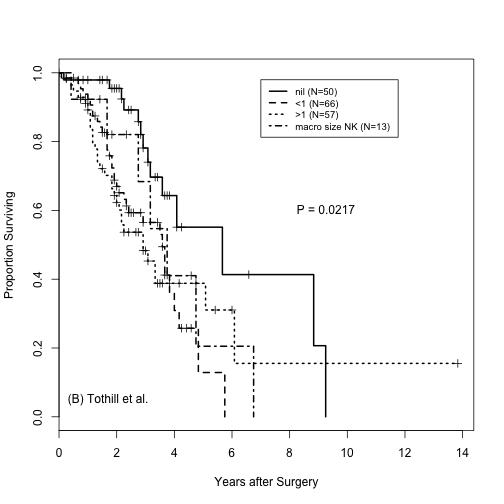For each data set, patients with any RD are compared to patients without RD. We do this first for the TCGA data.

``````
table(tcgaRDUse)
``````
``````## tcgaRDUse
## No RD    RD
##   113   378
``````
``````tcgaSurvFit <- survfit(survTCGA ~ tcgaRDUse)
survdiff(survTCGA ~ tcgaRDUse)
``````
``````## Call:
## survdiff(formula = survTCGA ~ tcgaRDUse)
##
##                   N Observed Expected (O-E)^2/E (O-E)^2/V
## tcgaRDUse=No RD 113       30     59.9     14.95      19.5
## tcgaRDUse=RD    378      231    201.1      4.46      19.5
##
##  Chisq= 19.5  on 1 degrees of freedom, p= 1e-05
``````
``````
plot(tcgaSurvFit, lty = 1:4, xlab = "Years after Surgery", ylab = "Proportion Surviving",
lwd = 2)
legend(x = 6, y = 0.98, legend = c("No RD (N=113)", "Any RD (N=378)"), lty = c(1:4),
lwd = 2, cex = 0.8)
text(0.05, 0.05, "(A) TCGA", pos = 4)
text(7, 0.6, "P < 0.0001", pos = 4)
``````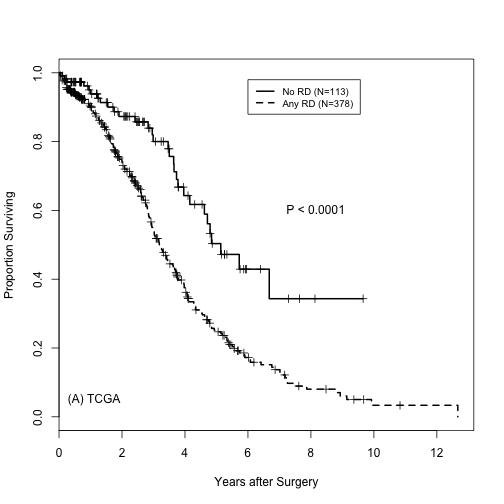We next do this for the data of Tothill et al.

``````
table(tothillRDUse)
``````
``````## tothillRDUse
## No RD    RD
##    50   136
``````
``````tothillSurvFit <- survfit(survTothill ~ tothillRDUse)
survdiff(survTothill ~ tothillRDUse)
``````
``````## Call:
## survdiff(formula = survTothill ~ tothillRDUse)
##
##                      N Observed Expected (O-E)^2/E (O-E)^2/V
## tothillRDUse=No RD  50       14       27      6.24      9.36
## tothillRDUse=RD    136       72       59      2.85      9.36
##
##  Chisq= 9.4  on 1 degrees of freedom, p= 0.00221
``````
``````
plot(tothillSurvFit, lty = 1:4, xlab = "Years", ylab = "Proportion Surviving",
lwd = 2)
legend(x = 6, y = 0.98, legend = c("No RD (N=50)", "Any RD (N=136)"), lty = c(1:4),
lwd = 2, cex = 0.8)
text(0.05, 0.05, "(B) Tothill et al.", pos = 4)
text(7, 0.6, "P  0.0022", pos = 4)
``````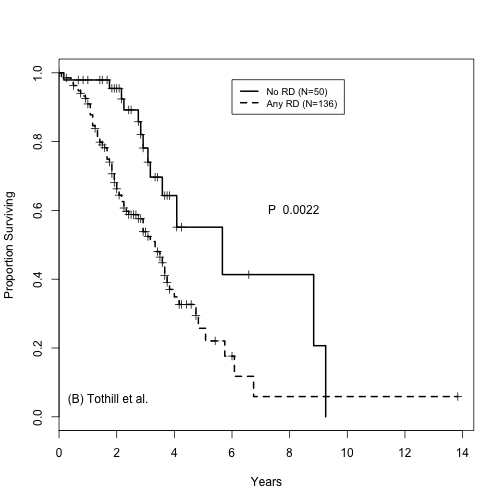We produce the TCGA plot for the manuscript.

``````
table(tcgaRDUse)
``````
``````## tcgaRDUse
## No RD    RD
##   113   378
``````
``````tcgaSurvFit <- survfit(survTCGA ~ tcgaRDUse)
survdiff(survTCGA ~ tcgaRDUse)
``````
``````## Call:
## survdiff(formula = survTCGA ~ tcgaRDUse)
##
##                   N Observed Expected (O-E)^2/E (O-E)^2/V
## tcgaRDUse=No RD 113       30     59.9     14.95      19.5
## tcgaRDUse=RD    378      231    201.1      4.46      19.5
##
##  Chisq= 19.5  on 1 degrees of freedom, p= 1e-05
``````
``````
plot(tcgaSurvFit, lty = 1:4, xlab = "Years after Surgery", ylab = "Proportion Surviving",
lwd = 2)
legend(x = 6, y = 0.98, legend = c("No RD (N=113)", "Any RD (N=378)"), lty = c(1:4),
lwd = 2, cex = 0.8)
text(0.05, 0.05, "(A) TCGA", pos = 4)
text(7, 0.6, "P < 0.001", pos = 4)
``````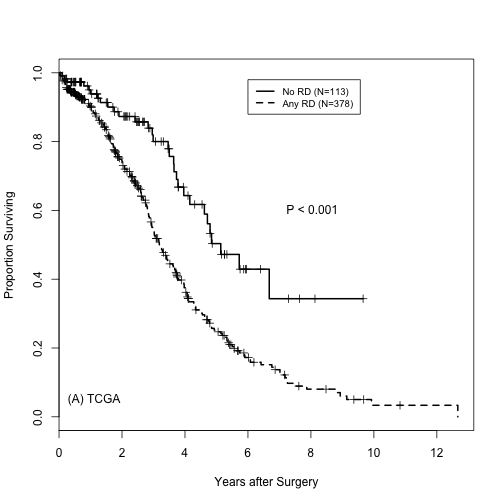We do the same thing for Tothill et al.

``````
table(tothillRDUse)
``````
``````## tothillRDUse
## No RD    RD
##    50   136
``````
``````tothillSurvFit <- survfit(survTothill ~ tothillRDUse)
survdiff(survTothill ~ tothillRDUse)
``````
``````## Call:
## survdiff(formula = survTothill ~ tothillRDUse)
##
##                      N Observed Expected (O-E)^2/E (O-E)^2/V
## tothillRDUse=No RD  50       14       27      6.24      9.36
## tothillRDUse=RD    136       72       59      2.85      9.36
##
##  Chisq= 9.4  on 1 degrees of freedom, p= 0.00221
``````
``````
plot(tothillSurvFit, lty = 1:4, xlab = "Years", ylab = "Proportion Surviving",
lwd = 2)
legend(x = 6, y = 0.98, legend = c("No RD (N=50)", "Any RD (N=136)"), lty = c(1:4),
lwd = 2, cex = 0.8)
text(0.05, 0.05, "(B) Tothill et al.", pos = 4)
text(7, 0.6, "P  0.002", pos = 4)
``````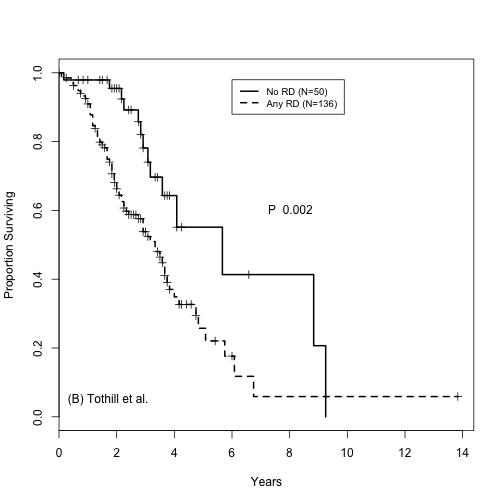We also look at OS in each data set for patients with FABP4 in the top 25% compared to the lower 75%. We begin with TCGA.

``````
probeNames <- rownames(tcgaExpressionUse)

library(hthgu133a.db)
``````
``````## Loading required package: AnnotationDbi
##
## Attaching package: 'BiocGenerics'
##
## The following objects are masked from 'package:parallel':
##
##     clusterApply, clusterApplyLB, clusterCall, clusterEvalQ,
##     clusterExport, clusterMap, parApply, parCapply, parLapply,
##     parLapplyLB, parRapply, parSapply, parSapplyLB
##
## The following object is masked from 'package:stats':
##
##     xtabs
##
## The following objects are masked from 'package:base':
##
##     anyDuplicated, as.data.frame, cbind, colnames, duplicated,
##     eval, Filter, Find, get, intersect, lapply, Map, mapply,
##     match, mget, order, paste, pmax, pmax.int, pmin, pmin.int,
##     Position, rank, rbind, Reduce, rep.int, rownames, sapply,
##     setdiff, sort, table, tapply, union, unique, unlist
##
## Welcome to Bioconductor
##
##     Vignettes contain introductory material; view with
##     'browseVignettes()'. To cite Bioconductor, see
##     'citation("Biobase")', and for packages 'citation("pkgname")'.
##
``````
``````geneNames <- unlist(mget(probeNames, hthgu133aSYMBOL))
probesFABP4 <- probeNames[which(geneNames == "FABP4")]
probesFABP4
``````
``````##  "203980_at"
``````
``````
tcgaFABP4 <- tcgaExpressionUse[probesFABP4, ]

tcgaFABP4Gp <- rep(0, length(tcgaFABP4))
tcgaFABP4Gp[tcgaFABP4 > quantile(tcgaFABP4, probs = c(0.75))] <- 1
table(tcgaFABP4Gp)
``````
``````## tcgaFABP4Gp
##   0   1
## 368 123
``````
``````
tcgaSurvFit <- survfit(survTCGA ~ tcgaFABP4Gp)
survdiff(survTCGA ~ tcgaFABP4Gp)
``````
``````## Call:
## survdiff(formula = survTCGA ~ tcgaFABP4Gp)
##
##                 N Observed Expected (O-E)^2/E (O-E)^2/V
## tcgaFABP4Gp=0 368      190    200.1     0.505      2.19
## tcgaFABP4Gp=1 123       71     60.9     1.659      2.19
##
##  Chisq= 2.2  on 1 degrees of freedom, p= 0.139
``````
``````
plot(tcgaSurvFit, lty = 1:4, xlab = "Years", ylab = "Proportion Surviving",
lwd = 2)
legend(x = 6, y = 0.98, legend = c("Low FABP4 (N=368)", "High FABP4 (N=123)"),
lty = c(1, 2), lwd = 2, cex = 0.8)
text(0.05, 0.05, "(A) TCGA", pos = 4)
text(7, 0.6, "P  0.139", pos = 4)
``````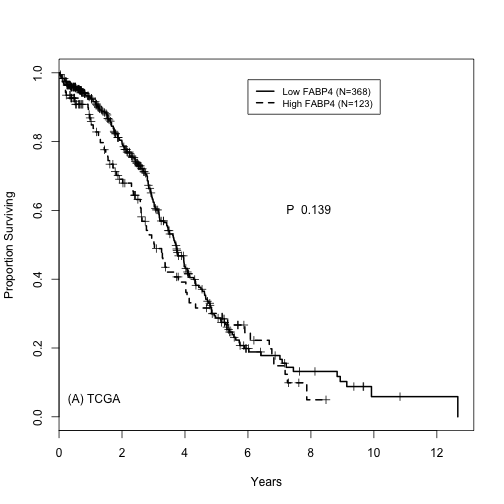We repeat, using the data of Tothill et al.

``````
tothillFABP4 <- tothillExpressionUse[probesFABP4, ]

tothillFABP4Gp <- rep(0, length(tothillFABP4))
tothillFABP4Gp[tothillFABP4 > quantile(tothillFABP4, probs = c(0.75))] <- 1
table(tothillFABP4Gp)
``````
``````## tothillFABP4Gp
##   0   1
## 139  47
``````
``````
tothillSurvFit <- survfit(survTothill ~ tothillFABP4Gp)
survdiff(survTothill ~ tothillFABP4Gp)
``````
``````## Call:
## survdiff(formula = survTothill ~ tothillFABP4Gp)
##
##                    N Observed Expected (O-E)^2/E (O-E)^2/V
## tothillFABP4Gp=0 139       56     67.4      1.92      9.17
## tothillFABP4Gp=1  47       30     18.6      6.97      9.17
##
##  Chisq= 9.2  on 1 degrees of freedom, p= 0.00246
``````
``````
plot(tothillSurvFit, lty = 1:2, xlab = "Years", ylab = "Proportion Surviving",
lwd = 2)
legend(x = 6, y = 0.98, legend = c("Low FABP4 (N=139)", "High FABP4 (N=47)"),
lty = c(1:2), lwd = 2, cex = 0.8)
text(0.05, 0.05, "(B) Tothill et al.", pos = 4)
text(7, 0.6, "P  0.0025", pos = 4)
``````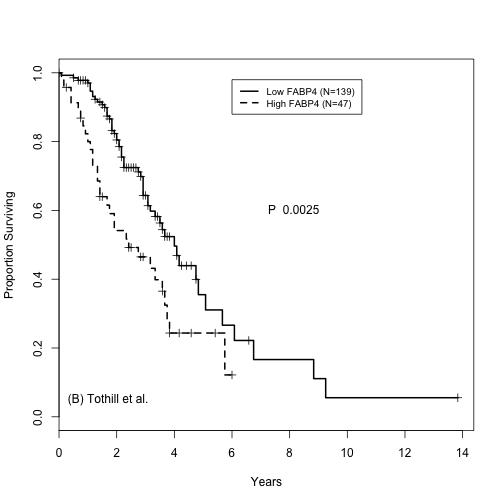## 4 Appendix

### 4.1 File Location

``````getwd()
``````
``````##  "/Users/slt/SLT WORKSPACE/EXEMPT/OVARIAN/Ovarian residual disease study 2012/RD manuscript/Web page for paper/Webpage"
``````

### 4.2 SessionInfo

``````sessionInfo()
``````
``````## R version 3.0.2 (2013-09-25)
## Platform: x86_64-apple-darwin10.8.0 (64-bit)
##
## locale:
##  en_US.UTF-8/en_US.UTF-8/en_US.UTF-8/C/en_US.UTF-8/en_US.UTF-8
##
## attached base packages:
##  parallel  splines   stats     graphics  grDevices utils     datasets
##  methods   base
##
## other attached packages:
##  hthgu133a.db_2.9.0   org.Hs.eg.db_2.9.0   RSQLite_0.11.4
##  DBI_0.2-7            AnnotationDbi_1.22.6 Biobase_2.20.1
##  BiocGenerics_0.6.0   survival_2.37-4      knitr_1.5
##
## loaded via a namespace (and not attached):
##  evaluate_0.5.1 formatR_0.9    IRanges_1.18.4 stats4_3.0.2
##  stringr_0.6.2  tools_3.0.2
``````